# Enthalpy

Enthalpy (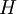$H$)  is defined as: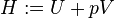$H:=U+pV$

where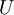$U$ is the internal energy,$p$ is the pressure,$V$ is the volume.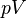$pV$ is a conjugate pair. The differential of this function is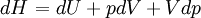$\left.dH\right.=dU+pdV+Vdp$

From the Second law of thermodynamics one obtains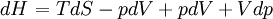$\left.dH\right.=TdS -pdV +pdV+Vdp$

thus we arrive at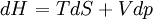$\left.dH\right.=TdS +Vdp$

For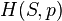$H(S,p)$ we have the following total differential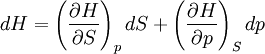$dH=\left(\frac{\partial H}{\partial S}\right)_p dS + \left(\frac{\partial H}{\partial p}\right)_S dp$